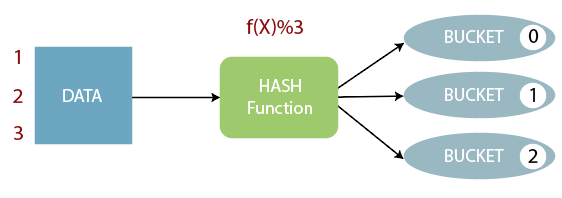# Bucketing in Hive

The bucketing in Hive is a data organizing technique. It is similar to partitioning in Hive with an added functionality that it divides large datasets into more manageable parts known as buckets. So, we can use bucketing in Hive when the implementation of partitioning becomes difficult. However, we can also divide partitions further in buckets.

## Working of Bucketing in Hive• The concept of bucketing is based on the hashing technique.
• Here, modules of current column value and the number of required buckets is calculated (let say, F(x) % 3).
• Now, based on the resulted value, the data is stored into the corresponding bucket.

### Example of Bucketing in Hive

• First, select the database in which we want to create a table.

hive> use showbucket;

• Create a dummy table to store the data.

hive> create table emp_demo (Id int, Name string , Salary float)
row format delimited
fields terminated by ‘,’ ;

• Now, load the data into the table.

hive> load data local inpath ‘/home/codegyani/hive/emp_details’ into table emp_demo;

• Enable the bucketing by using the following command: -

hive> set hive.enforce.bucketing = true;

• Create a bucketing table by using the following command: -
1. hive> create table emp_bucket(Id int, Name string , Salary float)
2. clustered by (Id) into 3 buckets
3. row format delimited
4. fields terminated by ‘,’ ;

• Now, insert the data of dummy table into the bucketed table.

hive> insert overwrite table emp_bucket select * from emp_demo;

• Here, we can see that the data is divided into three buckets.

• Let’s retrieve the data of bucket 0.

According to hash function :
6%3=0
3%3=0
So, these columns stored in bucket 0.

• Let’s retrieve the data of bucket 1.

According to hash function :
7%3=1
4%3=1
1%3=1
So, these columns stored in bucket 1.

• Let’s retrieve the data of bucket 2.

According to hash function :
8%3=2
5%3=2
2%3=2
So, these columns stored in bucket 2.